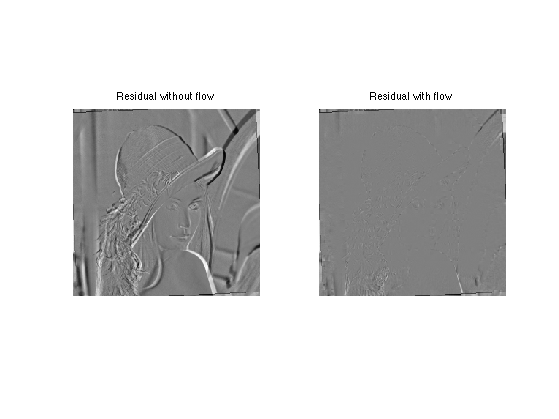$\newcommand{\NN}{\mathbb{N}} \newcommand{\CC}{\mathbb{C}} \newcommand{\GG}{\mathbb{G}} \newcommand{\LL}{\mathbb{L}} \newcommand{\PP}{\mathbb{P}} \newcommand{\QQ}{\mathbb{Q}} \newcommand{\RR}{\mathbb{R}} \newcommand{\VV}{\mathbb{V}} \newcommand{\ZZ}{\mathbb{Z}} \newcommand{\FF}{\mathbb{F}} \newcommand{\KK}{\mathbb{K}} \newcommand{\UU}{\mathbb{U}} \newcommand{\EE}{\mathbb{E}} \newcommand{\Aa}{\mathcal{A}} \newcommand{\Bb}{\mathcal{B}} \newcommand{\Cc}{\mathcal{C}} \newcommand{\Dd}{\mathcal{D}} \newcommand{\Ee}{\mathcal{E}} \newcommand{\Ff}{\mathcal{F}} \newcommand{\Gg}{\mathcal{G}} \newcommand{\Hh}{\mathcal{H}} \newcommand{\Ii}{\mathcal{I}} \newcommand{\Jj}{\mathcal{J}} \newcommand{\Kk}{\mathcal{K}} \newcommand{\Ll}{\mathcal{L}} \newcommand{\Mm}{\mathcal{M}} \newcommand{\Nn}{\mathcal{N}} \newcommand{\Oo}{\mathcal{O}} \newcommand{\Pp}{\mathcal{P}} \newcommand{\Qq}{\mathcal{Q}} \newcommand{\Rr}{\mathcal{R}} \newcommand{\Ss}{\mathcal{S}} \newcommand{\Tt}{\mathcal{T}} \newcommand{\Uu}{\mathcal{U}} \newcommand{\Vv}{\mathcal{V}} \newcommand{\Ww}{\mathcal{W}} \newcommand{\Xx}{\mathcal{X}} \newcommand{\Yy}{\mathcal{Y}} \newcommand{\Zz}{\mathcal{Z}} \newcommand{\al}{\alpha} \newcommand{\la}{\lambda} \newcommand{\ga}{\gamma} \newcommand{\Ga}{\Gamma} \newcommand{\La}{\Lambda} \newcommand{\Si}{\Sigma} \newcommand{\si}{\sigma} \newcommand{\be}{\beta} \newcommand{\de}{\delta} \newcommand{\De}{\Delta} \renewcommand{\phi}{\varphi} \renewcommand{\th}{\theta} \newcommand{\om}{\omega} \newcommand{\Om}{\Omega} \renewcommand{\epsilon}{\varepsilon} \newcommand{\Calpha}{\mathrm{C}^\al} \newcommand{\Cbeta}{\mathrm{C}^\be} \newcommand{\Cal}{\text{C}^\al} \newcommand{\Cdeux}{\text{C}^{2}} \newcommand{\Cun}{\text{C}^{1}} \newcommand{\Calt}{\text{C}^{#1}} \newcommand{\lun}{\ell^1} \newcommand{\ldeux}{\ell^2} \newcommand{\linf}{\ell^\infty} \newcommand{\ldeuxj}{{\ldeux_j}} \newcommand{\Lun}{\text{\upshape L}^1} \newcommand{\Ldeux}{\text{\upshape L}^2} \newcommand{\Lp}{\text{\upshape L}^p} \newcommand{\Lq}{\text{\upshape L}^q} \newcommand{\Linf}{\text{\upshape L}^\infty} \newcommand{\lzero}{\ell^0} \newcommand{\lp}{\ell^p} \renewcommand{\d}{\ins{d}} \newcommand{\Grad}{\text{Grad}} \newcommand{\grad}{\text{grad}} \renewcommand{\div}{\text{div}} \newcommand{\diag}{\text{diag}} \newcommand{\pd}{ \frac{ \partial #1}{\partial #2} } \newcommand{\pdd}{ \frac{ \partial^2 #1}{\partial #2^2} } \newcommand{\dotp}{\langle #1,\,#2\rangle} \newcommand{\norm}{|\!| #1 |\!|} \newcommand{\normi}{\norm{#1}_{\infty}} \newcommand{\normu}{\norm{#1}_{1}} \newcommand{\normz}{\norm{#1}_{0}} \newcommand{\abs}{\vert #1 \vert} \newcommand{\argmin}{\text{argmin}} \newcommand{\argmax}{\text{argmax}} \newcommand{\uargmin}{\underset{#1}{\argmin}\;} \newcommand{\uargmax}{\underset{#1}{\argmax}\;} \newcommand{\umin}{\underset{#1}{\min}\;} \newcommand{\umax}{\underset{#1}{\max}\;} \newcommand{\pa}{\left( #1 \right)} \newcommand{\choice}{ \left\{ \begin{array}{l} #1 \end{array} \right. } \newcommand{\enscond}{ \left\{ #1 \;:\; #2 \right\} } \newcommand{\qandq}{ \quad \text{and} \quad } \newcommand{\qqandqq}{ \qquad \text{and} \qquad } \newcommand{\qifq}{ \quad \text{if} \quad } \newcommand{\qqifqq}{ \qquad \text{if} \qquad } \newcommand{\qwhereq}{ \quad \text{where} \quad } \newcommand{\qqwhereqq}{ \qquad \text{where} \qquad } \newcommand{\qwithq}{ \quad \text{with} \quad } \newcommand{\qqwithqq}{ \qquad \text{with} \qquad } \newcommand{\qforq}{ \quad \text{for} \quad } \newcommand{\qqforqq}{ \qquad \text{for} \qquad } \newcommand{\qqsinceqq}{ \qquad \text{since} \qquad } \newcommand{\qsinceq}{ \quad \text{since} \quad } \newcommand{\qarrq}{\quad\Longrightarrow\quad} \newcommand{\qqarrqq}{\quad\Longrightarrow\quad} \newcommand{\qiffq}{\quad\Longleftrightarrow\quad} \newcommand{\qqiffqq}{\qquad\Longleftrightarrow\qquad} \newcommand{\qsubjq}{ \quad \text{subject to} \quad } \newcommand{\qqsubjqq}{ \qquad \text{subject to} \qquad }$

Optical Flow Computation

# Optical Flow Computation

This numerical tour explores the computation of optical flow between two images. It is at the heart of video coding.

## Installing toolboxes and setting up the path.

You need to download the following files: signal toolbox and general toolbox.

You need to unzip these toolboxes in your working directory, so that you have toolbox_signal and toolbox_general in your directory.

For Scilab user: you must replace the Matlab comment '%' by its Scilab counterpart '//'.

Recommandation: You should create a text file named for instance numericaltour.sce (in Scilab) or numericaltour.m (in Matlab) to write all the Scilab/Matlab command you want to execute. Then, simply run exec('numericaltour.sce'); (in Scilab) or numericaltour; (in Matlab) to run the commands.

Execute this line only if you are using Matlab.

getd = @(p)path(p,path); % scilab users must *not* execute this


Then you can add the toolboxes to the path.

getd('toolbox_signal/');
getd('toolbox_general/');


To evaluate the performance of optical flow computation, we compute a pair of image obtained by a smooth warping (small deformation), here a simple rotation.

Original frame #1.

n = 256;
name = 'lena';


The second image M2 is obtaind by rotating the first one.

% angle of rotation
theta = .03 * pi/2;
% original coordinates
[Y,X] = meshgrid(1:n,1:n);
% rotated coordinates
X1 = (X-n/2)*cos(theta) + (Y-n/2)*sin(theta) + n/2;
Y1 =-(X-n/2)*sin(theta) + (Y-n/2)*cos(theta) + n/2;
% boundary handling
X1 = mod(X1-1,n)+1;
Y1 = mod(Y1-1,n)+1;
% interpolation
M2 = interp2(Y,X,M1,Y1,X1);
M2(isnan(M2)) = 0;


Display the two images.

clf;
imageplot(M1, 'Frame #1', 1,2,1);
imageplot(M2, 'Frame #2', 1,2,2);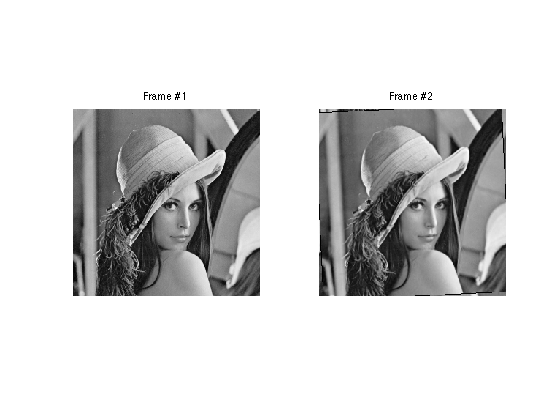## Optical Flow Computation with Regularization

A first approach to optical flow computation is to solve a ill posed problem corresponding to the optical flow equation constraint (consistency of gray level intensity when moving along the flow).

Compute the derivatives in time and space.

global D;
Dt = M1-M2;

Warning: The value of local variables may have been changed to match the
globals.  Future versions of MATLAB will require that you declare a variable to
be global before you use that variable.


Display them.

clf;
imageplot(Dt, 'd/dt', 1,3,1);
imageplot(D(:,:,1), 'd/dx', 1,3,2);
imageplot(D(:,:,2), 'd/dy', 1,3,3);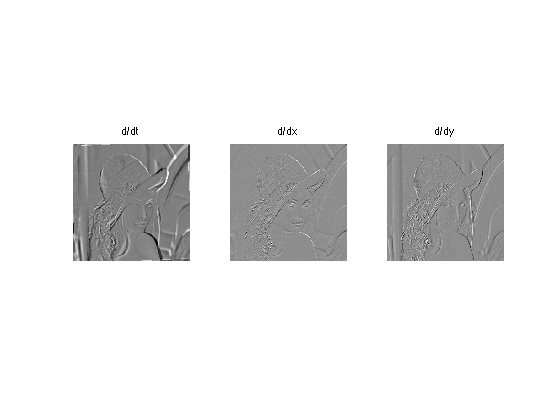The optical flow constraint asks for consistency of gray levels when moving along the flow v=[v1,v2]. This is written as a linear equation

Dt + v1.*D1 + v2.*D2=0

This equation does not constrain enough the flow (one equation for two unknown). One thus needs to add other constraints, and this is achieved by performing a Sobolev regularization, as first proposed by Horn and Schunck in the paper:

Horn, B.K.P., and Schunck, B.G., Determining Optical Flow, AI(17), No. 1-3, August 1981, pp. 185-203

This corresponds to a quadratic regularization with a Sobolev prior:

Its solution is computed by solving a linear system resolution, which sets to zero the gradient of the functional. It can be computed using a gradient descent, or, better, a conjugate gradient descent. We first detail the gradient descent, and shows that is not very efficient.

Regularization strength.

global lambda;
lambda = .1;

Warning: The value of local variables may have been changed to match the
globals.  Future versions of MATLAB will require that you declare a variable to
be global before you use that variable.


tau = .2;


Initialization.

v = zeros(n,n,2);


Compute the gradient of the functional. First compute Dt+v1*D1+v2*D2

U = Dt + sum(v.*D,3);


Then compute the Laplacian L(:,:,k) of each channel v(:,:,k) of the vector field

L = cat(3, div(grad(v(:,:,1))), div(grad(v(:,:,2))));


And gather everything together to build the gradient of the functional.

G = D.*repmat(U, [1 1 2])  - lambda * L;


Perform the descent.

v = v - tau*G;


Exercice 1: (check the solution) Perform the gradient descent of the energy, and display the decay of the energy.

exo1;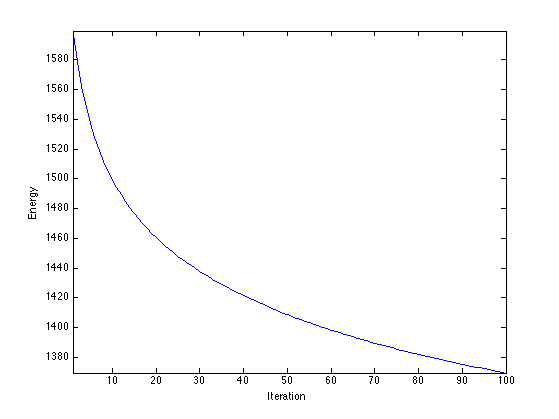A much faster algorithm is the conjugate gradient. Several variant are implemented within matlab, and can be used by implementing a callback function.

Set up parameters for the CG algorithm (tolerance and maximum number of iterations.

tol = 1e-5;
maxit = 200;


Right hand side of the linear system.

b = -D.*cat(3,Dt,Dt);


[v,flag,relres,it,resvec] = cgs(@callback_optical_flow,b(:),tol,maxit);
v = reshape(v, [n n 2]);


Display the flow as a color image and as arrows.

clf;
imageplot(v, '', 1,2,1);
subplot(1,2,2);
w = 12; m = ceil(n/w);
t = w/2 + ((0:m-1)*w);
[V,U] = meshgrid(t,t);
hold on;
imageplot(M1);
quiver(t,t,v(1:w:n,1:w:n,2), v(1:w:n,1:w:n,1));
axis('ij');Compute the image warped along the flow.

% compute the grid, translated along the flow
[Y,X] = meshgrid(1:n,1:n);
X = clamp(X+v(:,:,1),1,n);
Y = clamp(Y+v(:,:,2),1,n);
% compute the first fame, translated along the flow
Ms = interp2( 1:n,1:n, M1, Y,X );


One can compare the residual with and without the flow

% residual without flow
R0 = M2-M1;
% residual along the flow
R = Ms-M1;
% ensure same dynamic range (just for display)
v = max( [max(abs(R0(:))) max(abs(R(:)))] );
R(1)=v; R(2)=-v; R0(1)=v; R0(2)=-v;
% display
clf;
imageplot(R0, 'Residual without flow', 1,2,1);
imageplot(R, 'Residual with flow', 1,2,2);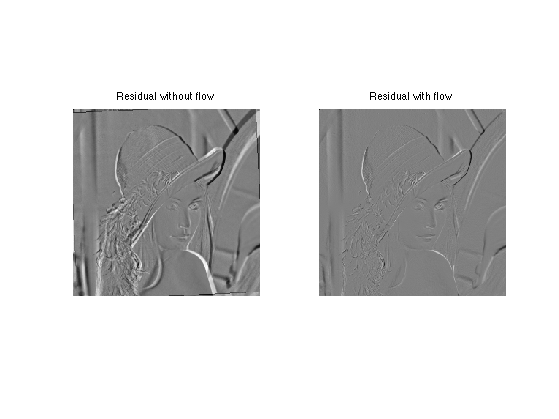## Optical Flow Computation with Block Matching

A second approach to compute the optical flow is to perform local block matching, as first proposed by Lucas and Kanade in

Lucas B D and Kanade T, An iterative image registration technique with an application to stereo vision Proceedings of Imaging understanding workshop, pp 121-130, 1981.

The advantage is that this is more precise than the global Horn/Schunck method, and it might also be faster (no iterative scheme is needed). The desadvantage is that it does not regularize the flow in flat region.

% An optical flow is a vector field that describes
% the movement between to
% consecutive frames of the video.


The flow can be computed by block matching. A block of (2*k+1,2*k+1) pixels in frame 1 around a location (x,y) is compared to the blocks at locations (x+dx,y+dy) for -q<=dy,dx<=q in the frame 2.

% width of the block
w = 8;
% search width
q = 4;
% sub-pixelic search if <1
dq = .5;


Number of flow vector is m^2.

m = ceil(n/w);


Precompute movements vectors.

[X0,Y0,dX,dY] = ndgrid( 0:w-1, 0:w-1, -q:dq:q,-q:dq:q);
[dy,dx] = meshgrid(-q:dq:q,-q:dq:q);


Start with empty optical flow. Each f=F(x,y,:) is a 2D vector mapping the patch at location (x,y) to the patch (x+f(1),y+f(2).

F = zeros(n,n,2);


Example of block number for wich the flow is computed. Each index should be less than m

i = 3; j = 40;


Pixel numbers.

x = (i-1)*w+1;
y = (j-1)*w+1;


Block pixels index.

selx = clamp( (i-1)*w+1:i*w, 1,n);
sely = clamp( (j-1)*w+1:j*w, 1,n);


A special care should be taken at the boundary : we simply clamp values outside boundaries

X = clamp(x + X0 + dX,1,n);
Y = clamp(y + Y0 + dY,1,n);


Compute base patch of M2 at which the flow is computed.

P2 = M2(selx,sely);


Compute patches of M1 that are matched. Use interpolation to handle non indeger pixel indexes.

P1 = interp2( 1:n,1:n, M1, Y,X );


Compute the distance between P1 and all the patches of P2.

d = sum(sum( (P1-repmat(P2,[1 1 size(P1,3) size(P1,4)])).^2 ) );


Compute best match and report its value.

[tmp,I] = compute_min(d(:));
F(selx,sely,1) = dx(I);
F(selx,sely,2) = dy(I);


Exercice 2: (check the solution) Compute the whole optical flow F, by cycling through the pixels.

exo2;


Display the flow as a color image and as arrows.

clf;
imageplot(F, '', 1,2,1);
subplot(1,2,2);
t = w/2 + ((0:m-1)*w);
[V,U] = meshgrid(t,t);
hold on;
imageplot(M1);
quiver(t,t,F(1:w:n,1:w:n,2), F(1:w:n,1:w:n,1));
axis('ij');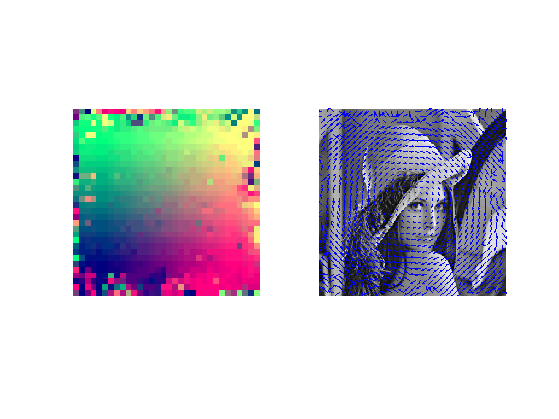## Residual Computation

The optical flow F allows one to compute the residual R between frame M2 and an extrapolated version of M1 along the flow F.

One can translate the first frame M1 along the flow F.

% compute the grid, translated along the flow
[Y,X] = meshgrid(1:n,1:n);
X = clamp(X+F(:,:,1),1,n);
Y = clamp(Y+F(:,:,2),1,n);
% compute the first fame, translated along the flow
Ms = interp2( 1:n,1:n, M1, Y,X );


One can compare the residual with and without the flow

% residual without flow
R0 = M2-M1;
% residual along the flow
R = M2-Ms;
% ensure same dynamic range (just for display)
v = max( [max(abs(R0(:))) max(abs(R(:)))] );
R(1)=v; R(2)=-v; R0(1)=v; R0(2)=-v;
% display
clf;
imageplot(R0, 'Residual without flow', 1,2,1);
imageplot(R, 'Residual with flow', 1,2,2);